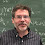## Sunday, June 01, 2014

### Law of small numbers and letters

The law of small numbers is that there are not enough small numbers for all of the tasks that are assigned to them. That makes some math cranks find patterns which are really only caused by not enough small numbers around. One such crank kept finding the number 57 in events related to the American Revolutionary war. The fallacy is that if you look hard enough ANY number would work.

At the LLL workshop it was noted that LLL stands for both Local Lovasz Lemma (my wife asked an entire workshop about a lemma?'') or the Lensta-Lenstra-Lovasz algorithm. Here we see that there aren't quite enough sequences of letters, hence some get used twice. Of course in this case it helps that Lovasz is brilliant.

The only other example I now of two well known theorems with the same initials of authors is AKS:

AKS primality testing: Agrawal-Kayal-Sazena test of primality in poly time

and

AKS sorting network: Ajtai-Komlos-Szemeredi  O(log n) sorting network.

AKS primality gets 13,500 hits

AKS sorting network gets 39,100 hits

Are there other pairs of well known results where the initials are the same?
(This may depend on what you call well known'')

When someone says AKS do you think primality or sorting network?
It may depend on your age and your field. I think of the Ajtai-Komlos-Szemeredi upper bound R(3,k) \le O(k^2/log(k)).  I tend to NOT count that as another collision of initials since its the exact same authors as the other paper.

1.PCP: Post Correspondence Problem or Probabilistically Checkable Proofs?

2.A collision that I discovered when I checked what talks were up:

PCP=Probabilistically Checkable Proof
PCP=Post Correspondence Problem

3.There is also a recent paper by An-Kleinberg-Shmoys on the TSP path problem.

4.PCP seems like a good example of an acronym that can mean two very different things, both widely known in computer science (and without any common author).

5.Also AKS = Ajtai-Kumar-Sivakumar, the first singly exponential (2^n) algorithm for the Shortest Vector Problem in n-dimensional lattices.

Bottom line: if you review a paper/proposal by AKS, accept it.

6.For AKS, I don't think about either of your two choices, because it's "Komlos", not "Kolmos". More precisely, it's "Komlós".

1.Fixed to Komlos.. Thanks.

7.AKS primes = 122000 hits.

8.I thought AKS is used for referring to extremely difficult to understand algorithms and is abbreviating a German expression like "algorithmus klieg schwierig" (not sure about K).

9.Another example, when an acronym is used in quite a few unrelated fields:

ATM = Alternating Turing Machine
ATM = Asynchronous Transfer Mode
ATM = Automated Teller Machine
ATM = Air Traffic Management
ATM = Anti-Tank Missile
ATM = Area Training Manager
ATM = Automatic Timing Mechanism
ATM = Atmospheric Transport Model
ATM = Air Turbine Motor
ATM = Association of Texas Midwives
ATM = Acoustic Telemetry Modem
ATM = Automated Theorem Prover

and probably a number of others...

1.If you're going to go there then there's the obvious one that makes certain complexity theorists sound like drug dealers (which also happens to collide with an idea in computability as pointed out by two Anons above)

10.Definitely AKS makes me think primes, not sorting network. Similarly PCP = probabilistically checkable proof.

11.Actually, TSP is a good example.

Travelling Salesman Problem
Time Stamp Protocol
Telecommunications Service Provider